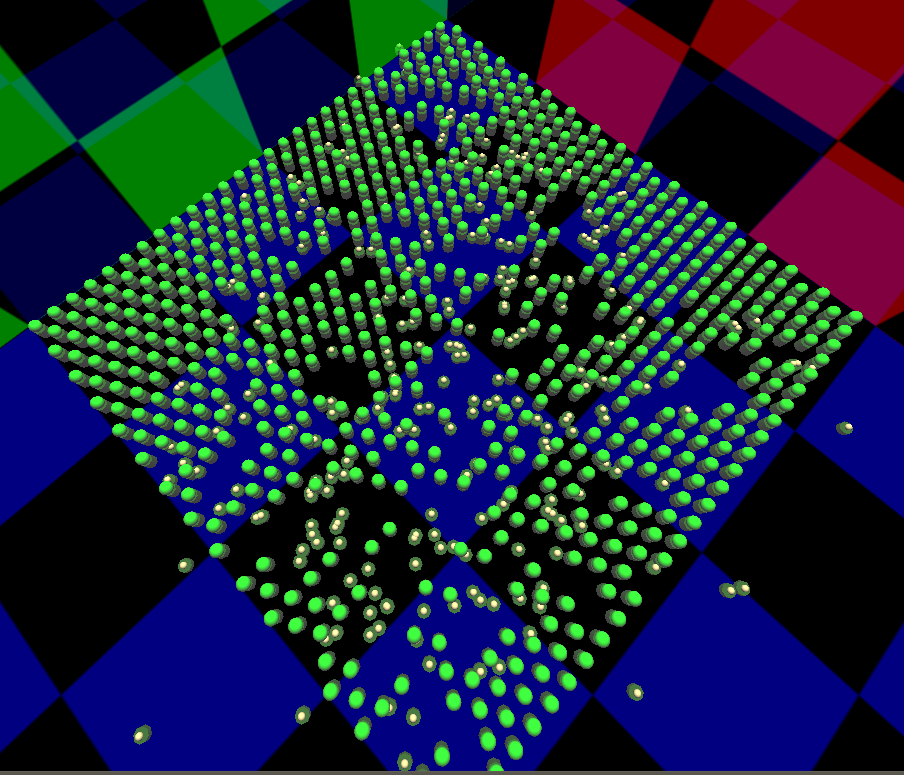# fission

## Nuclear fission - chain reaction

ModelId:
fission4.sml
SimileVersion:
6.6p2

This model simulates the process of nuclear fission by chain reaction, such as occurs in a fission reactor or weapon. It models a sample of a fissile material, such as plutonium, which has the property that when a nucleus is hit by a neutron it splits, releasing two more neutrons which are capable of splitting other nuclei. In a fission reactor this process is continuous, and regulated by the insertion of rods of a material that absorbs neutrons, in order to release a controllable amount of energy.

Equations:

Model Desktop1 :

Event   start :
start =         1 (boolean)
Maximum = 0
Event that occurs once at start of simulation

State   split id :
split id =         [[U235 atoms/fission,trigger_magnitude()],[reset...,0]] (real)

Only one atom should split at a time, so this state is the index of the last atom to split

Submodel Hit :

Submodel "Hit" an association submodel between "U235 atoms" (in role "is") and "Neutrons" (in role "does").

Condition   cond1 :
cond1 =         dist<1 (cond_spec)
Where:
dist = Value(s) of dist_sqd

True if neutron and atom are less than 1 distance unit apart. This allows the time of the collision event to be set precisely since the association will already exist.

Event   happens :
happens =         dist_sqd (boolean)
Where:
dist_sqd = Value(s) of dist_sqd
Minimum = 0.25
Splitting happens if neutron gets within 0.5 distance units of atom.

Variable   dist_sqd :
dist_sqd =         (is_x-does_x)^2+(is_y-does_y)^2 (real)
Where:
is_y = Value(s) of ../U235 atoms/y from submodel "U235 atoms" in role "is"
{every_y} = Value(s) of ../U235 atoms/y
is_x = Value(s) of ../U235 atoms/x from submodel "U235 atoms" in role "is"
{every_x} = Value(s) of ../U235 atoms/x
does_x = Value(s) of ../Neutrons/x from submodel "Neutrons" in role "does"
{every_x_0} = Value(s) of ../Neutrons/x
does_y = Value(s) of ../Neutrons/y from submodel "Neutrons" in role "does"
{every_y_0} = Value(s) of ../Neutrons/y

Square of distance between neutron and atom

Submodel U235 atoms :

Submodel "U235 atoms" is a population submodel.

Creation   cr1 :
cr1 =         896 (real)

Initial population of atoms

Event   decay :
decay =         after(exprnd(10000),"true") (boolean)

Each atom will spontaneously decay after a time sampled from a random-exponential distribution if left alone

Event   fission :
fission =         index(1) (real)

Caused either by spontaneous decay or splitting by a neutron. Value is index of atom.

Loss   loss1 :
loss1 =         1 (int)

Atom always removed after fission occurs

Variable   size :
size =         2 (int)

For lollipop display

Variable   x :
x =         element([x],index(1)) (real)
Where:
[x] = Value(s) of ../start posns/x

Variable   y :
y =         element([y],index(1)) (real)
Where:
[y] = Value(s) of ../start posns/y

Submodel Neutrons :

Submodel "Neutrons" is a population submodel.

Compartment   x :
Initial value = element([x],split_id) (real)
Where:
[x] = Value(s) of ../start posns/x
split_id = Value(s) of ../split id

Starting X position, looked up from array using index of atom that just split

Compartment   y :
Initial value = element([y],split_id) (real)
Where:
[y] = Value(s) of ../start posns/y
split_id = Value(s) of ../split id

Starting Y position, looked up from array using index of atom that just split

Flow   flow1 :
flow1 =         rand_const(-0.5,0.5) (1/day)

Flow   flow2 :
flow2 =         rand_const(-0.5,0.5) (1/day)

Immigration   im1 :
im1 =         2 (real)

Number of new neutrons produced when an atom undergoes fission

Loss   loss102 :
loss102 =         x<-20 or x>120 or y<-20 or y>120 (boolean)
Where:
x = Value(s) of x
y = Value(s) of y

Neutron is removed from simulation if it is heading away from the atoms to save processing time

Loss   loss2 :
loss2 =         1 (int)

Old neutron removed when it splits an atom, so new ones will have new random trajectories

Variable   size :
size =         1 (int)

For lollipop display

Submodel start posns :

Submodel "start posns" is a fixed_membership multi-instance submodel with dimensions .
This is a 1-D array for calculating the positions of all the atoms, used to set them up and to set positions of new neutrons when they split

Variable   x :
x =         3*((index(1)-1)%32)+3 (real)

Variable   y :
y =         3.464*ceil(index(1)/32)+1.732*(index(1)%2) (real)

Results:

This image is a screenshot taken while executing the model in SimiLive. In some areas the atom grid is complete, while in others many atoms (green) are missing and there are large numbers of neutrons (orange).Model tags: